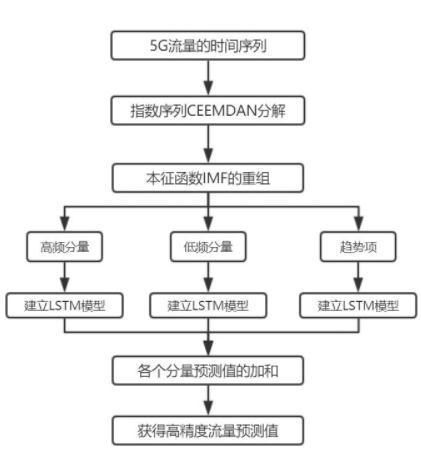## 简历项目经验1：CEEMDAN-LSTM 5G基站流量预测

2021-08-15
0 评论 0 浏览

CEEMDAN-LSTM 5G 基站流量预测

## CEEMDAN

EMD
2000 年以来，有很多突破性的频谱分析方法， EMD［13－14］方法是大家都认可的一种新型方法，此方法主要描述数据本身，不需要安插其他基函数，只需要按照数据本身的时间尺度特征进行信号方面的分解。这也正是它与其他方法的差异所在。正是由于 EMD 拥有这样独特的性质，所以在理论层面上各类信号都可以用 EMD 处理，尤其是在处理非线性数据上，EMD 拥有很明显的优势，优胜于其他方法，并且具备很高的信噪比。自从提出 EMD 方法之后，各个领域都广泛应用其处理一些棘手的问题数据，比如空气质量、海洋数据、天体观测数据资料分析、地震记录数据分析。EMD 的主要工作就是将数据分解成不同尺度的各个分量，也称其为 IMF，通过 IMF 可以更加直观地观测数据。

EMD 本质是经过特征的时间尺度识别信号中所含有的所有振动模态。在 EMD 分解中，每个 IMF 的状态都具有一定的相同性，每个 IMF 都具有信号的某一时间尺度特征。相比于其他的信号分析方法，EMD 分解具有很大可行优势，它的基函数是由自身得到的，所以能在各类的信号处理方面游刃有余，没有特别严格的限定，且具有普遍性。

EMD 的优势如下:
(1) EMD 分解的真正运行是将原数据分解成频率不同的各个数据，由高到低依次得到。对于各个种类的信号来说，高频数据往往代表着此数据的主要特征，所以 EMD 是一种将高频分量先行提出的一种方法，从另一角度说也是一种新的主成分分析法。

(2) EMD 能根据分解过程中的信号特征自适应发生改变，所以 EMD 方法具有自适应时序分析特征。算法如下：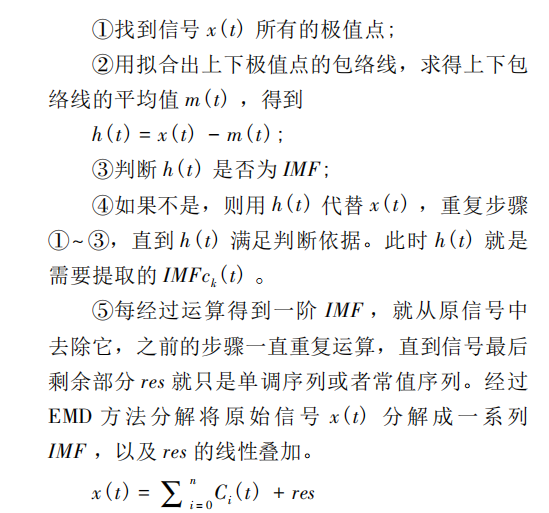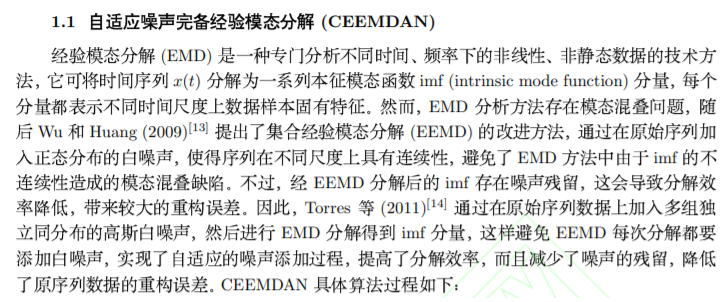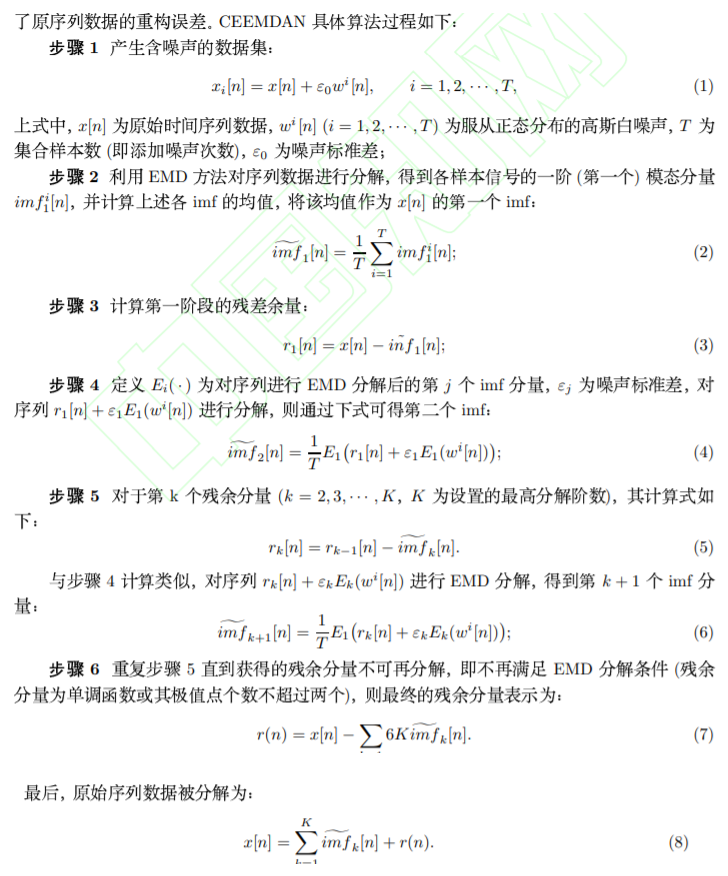``````% Example of the CEEMDAN performance, used in the work where CEEMDAN was first presented:
% Cite:
% M.E.TORRES, M.A. COLOMINAS, G. SCHLOTTHAUER, P. FLANDRIN,
%  "A complete Ensemble Empirical Mode decomposition with adaptive noise,"
%  IEEE Int. Conf. on Acoust., Speech and Signal Proc. ICASSP-11, pp. 4144-4147, Prague (CZ)
%
% The code loads the signal ecg.mat
% It is an ECG from the MIT-BIH Normal Sinus Rhythm Database
% available at http://www.physionet.org/cgi-bin/atm/ATM.
% The first 10 seconds of the first channel of the record 16265 has been
% used in the aboved mentioned paper
%
% -------------------------------------------------------------------------
% Date: June 06,2011
% Authors:  Torres ME, Colominas MA, Schlotthauer G, Flandrin P.
% To:  macolominas(AT)bioingenieria.edu.ar
% CC:  metorres(AT)santafe-conicet.gov.ar
% -------------------------------------------------------------------------
%  This version was run on Matlab 7.10.0 (R2010a)
%--------------------------------------------------------------------------

%m=aaa(1:1000,1);
m=aaa(:,1);
ecg1=m';

Nstd = 0.2;
NR = 500;
MaxIter = 5000;

[modes its]=ceemdan(ecg1,0.2,500,5000);
t=1+1853:length(ecg1)+1853;

[a b]=size(modes);

figure;
subplot(a+1,1,1);
plot(t,ecg1);
ylabel('ECG')
set(gca,'xtick',[])
axis tight;

for i=2:a
subplot(a+1,1,i);
plot(t,modes(i-1,:));
ylabel (['IMF ' num2str(i-1)]);
set(gca,'xtick',[ ])
xlim([1+1853 length(ecg1)+1+1853])
end;

subplot(a+1,1,a+1)
plot(t,modes(a,:))
ylabel(['IMF ' num2str(a)])
xlim([1+1853 length(ecg1)+1853])

figure;
boxplot(its);

for j=1:7
pp=modes(j,:);
ttest(pp)
end
%%
mmm=modes(1,:)+modes(2,:)+modes(4,:)+modes(3,:)+modes(5,:);%高频分量
nnn=modes(6,:)+modes(7,:);%低频分量

figure
subplot(3,1,1)
plot(1+1949:69+1949,mmm)
subplot(3,1,2)
plot(1+1949:69+1949,nnn)
subplot(3,1,3)
plot(1+1949:69+1949,modes(7,:))

mm2=mmm';
nn2=nnn';
rr2=modes(7,:)';

``````

## LSTM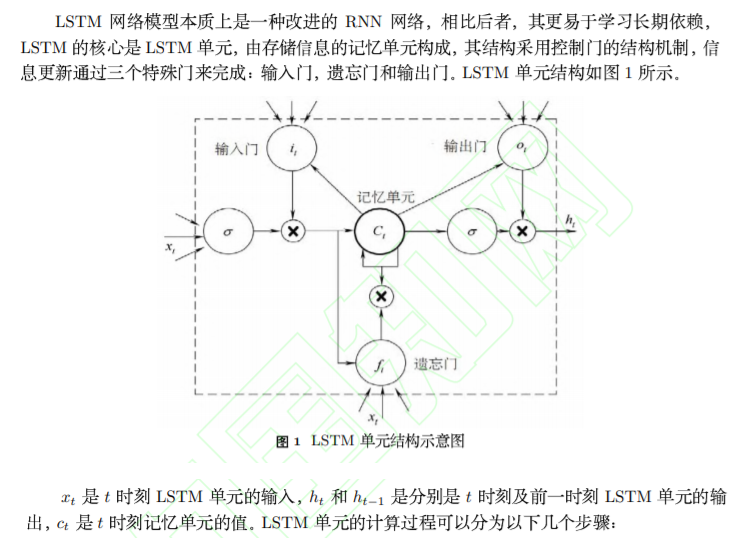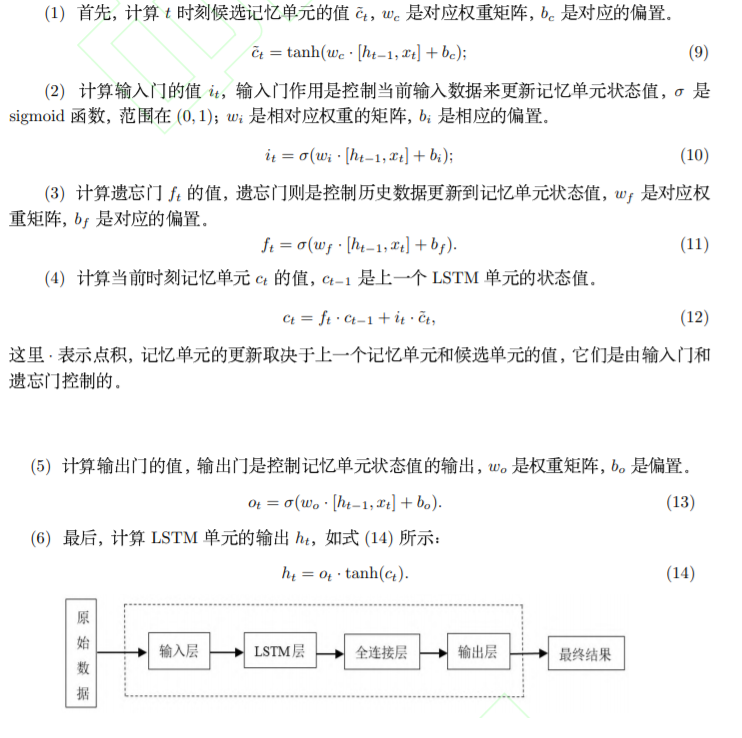``````#定义滚动函数
from numpy import array
from keras.models import Sequential
from keras.layers import LSTM
from keras.layers import Dense

'''

'''
# split a univariate sequence into samples
def split_sequence(sequence, n_steps):
X, y = list(), list()
for i in range(len(sequence)):
# find the end of this pattern
end_ix = i + n_steps
# check if we are beyond the sequence
if end_ix > len(sequence)-1:
break
# gather input and output parts of the pattern
seq_x, seq_y = sequence[i:end_ix], sequence[end_ix]
X.append(seq_x)
y.append(seq_y)
return array(X), array(y)

if __name__ == '__main__':

# define input sequence
raw_seq = nd_s12
print (raw_seq)
# choose a number of time steps
n_steps = 30
# split into samples
X, y = split_sequence(raw_seq, n_steps)
print (X, y)
# reshape from [samples, timesteps] into [samples, timesteps, features]
n_features = 1
X = X.reshape((X.shape, X.shape, n_features))
# define model
model = Sequential()
model.add(LSTM(50, activation='relu', input_shape=(n_steps, n_features)))     # 隐藏层，输入，特征维
# fit model 拟合
model.fit(X, y, epochs=300, batch_size=1, verbose=2)  # 迭代次数，批次数，verbose决定是否显示每次迭代
``````